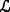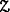$$\tilde {c}$$(9, 11) 10
$$\tilde {s}_{1}$$(5, 7) 6
$$\tilde {s}_{2}$$(4, 6) 5
$$\tilde {d}_{1}$$(2900, 3000, 3300) 3050
$$\tilde {d}_{2}$$(2800, 3000, 3100) 2975
$$\tilde {\beta }$$(80, 120) 100
$$\tilde {\gamma }$$(40, 60) 50